## Logistic Regression 模型简介

600010人浏览 / 2015-05-08 00:00:00.0

## 逻辑回归

### 问题

1. 预测一个用户是否点击特定的商品
2. 判断用户的性别
3. 预测用户是否会购买给定的品类
4. 判断一条评论是正面的还是负面的

$$D = {(x^1, y^1), (x^2, y^2) … (x^N, y^N)}$$

### 模型

#### sigmoid 函数

$$g(x) = \frac{1}{1 + e ^ {-x}}$$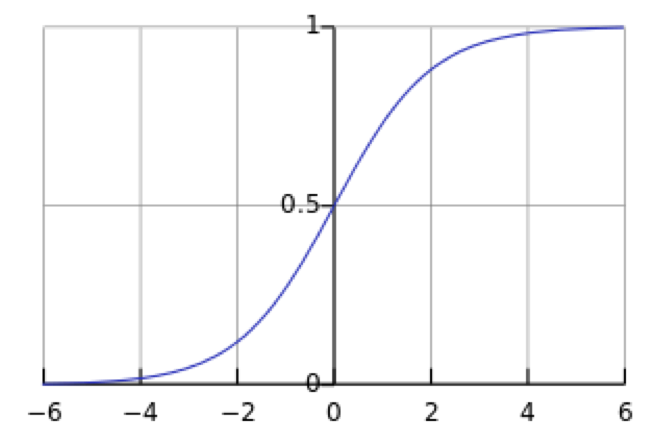#### 决策函数

$$P(y=1|x;\theta) = g(\theta^T x) = \frac{1}{1 + e ^ {-\theta^T * x}}$$

$$y^* = 1, \, \textrm{if} \, P(y=1|x) > 0.5$$

#### 参数求解

$$L(\theta) = P(D|\theta) = \prod P(y|x;\theta) = \prod g(\theta^T x) ^ y (1-g(\theta^T x))^{1-y}$$

$$l(\theta) = \sum {y\log{g(\theta^T x)} + (1-y)\log{(1-g(\theta^T x))}}$$

$$J(\theta) = -\frac{1}{N} l(\theta)$$

• 选择下降方向（梯度方向，$$\nabla {J(\theta)}$$）
• 选择步长，更新参数 $$\theta^i = \theta^{i-1} - \alpha^i \nabla {J(\theta^{i-1})}$$
• 重复以上两步直到满足终止条件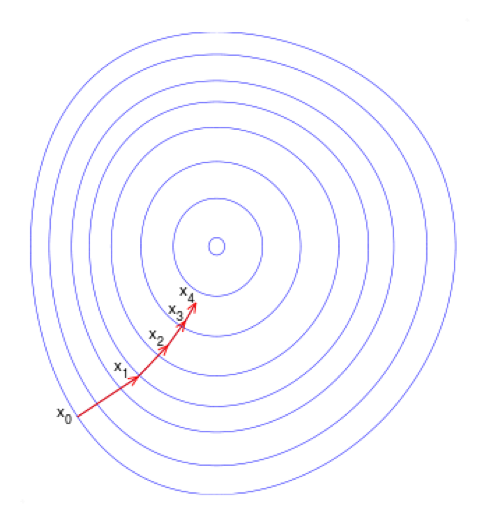$$\frac{\partial{J}}{\partial{\theta}} = -\frac{1}{n}\sum_i (y_i - y_i^*)x_i + \lambda \theta$$

#### 分类边界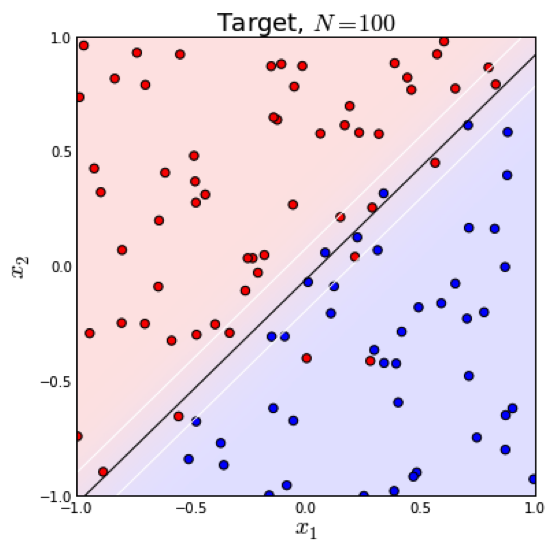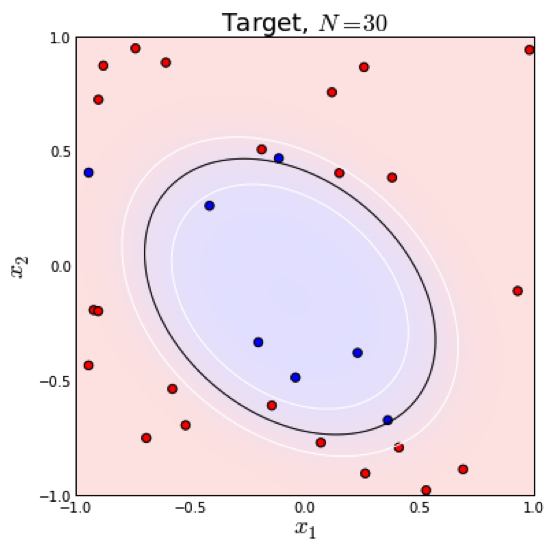#### 正则化

$$J(\theta) = -\frac{1}{N}\sum {y\log{g(\theta^T x)} + (1-y)\log{(1-g(\theta^T x))}} + \lambda \Vert w \Vert_p$$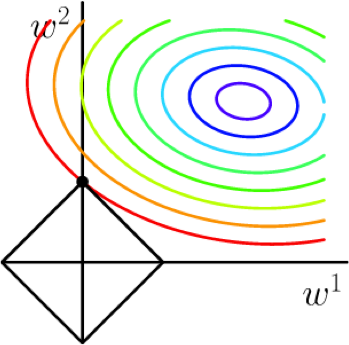## 延伸

### 生成模型和判别模型

$$p(y|x) = \frac{P(x|y)P(y)}{\sum{P(x|y)P(y)}}$$

$$\log\frac{P(y=1|x)}{P(y=0|x)} = \log\frac{P(x|y=1)}{P(x|y=0)} + \log\frac{P(y=1)}{P(y=0)} = -\frac{(x-\mu_1)^2}{2\sigma_1^2} + \frac{(x-\mu_0)^2}{2\sigma_0^2}\ + \theta_0$$

$$\log\frac{P(y=1|x)}{P(y=0|x)} = \theta^T x$$

$$P(y=1|x) = \frac{e^{\theta^T x}}{1+e^{\theta^T x}} = \frac{1}{1+e^{-\theta^T x}}$$

### 多分类（softmax)

$$P(y=i|x, \theta) = \frac{e^{\theta_i^T x}}{\sum_j^K{e^{\theta_j^T x}}}$$

$$J(\theta) = -\frac{1}{N} \sum_i^N \sum_j^K {1[y_i=j] \log{\frac{e^{\theta_i^T x}}{\sum {e^{\theta_k^T x}}}}}$$

## 参考资料

• Trevor Hastie et al. The elements of statistical learning
• Andrew Ng, CS 229 lecture notes
• C.M. Bishop, Pattern recognition and machine learning
• Andrew Ng et al. On discriminative vs. generative classifiers:a comparison of logistic regression and naïve bayes
• Wikipedia, http://en.wikipedia.org/wiki/Logistic_regression

#### 相关文章